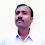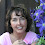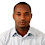### Books in a Library

In a library, 30% books are English books and 10% are Hindi books. If the remaining 3600 books are in Malayalam.

What is the total number of books in that library?

1.Nice and educative blog.

2.3.4.100-30-10=60
3600/60=60
60*100=6000

5.6.7.8.for 60%---3600books
then40%---?
on cross multiplication=2400
hence total no. of books=3600+2400=6000

9.6000 books

10.6000 books

11.100x3600/60=6000
a cool and educating blog.

12.6000 books

13.6000 books

14.15.16.6000 Books,
60% of Books = 3600
30% of Books = 1800 (Half of above)
10% of Books = 600 (1/3 of above)

Total Books 6000

17.3600 = 60%
2400 = 40%
Total = 6000 books

18.This comment has been removed by the author.

1.19.20.21.22.6000 books. 30+10=40%......Therefore 60% remains. 3600 is 60% of what? 3600/.60=6000 books.

23.24.6000 books...

25.This comment has been removed by the author.

26.27.28.6000-40% =3600.
6000-30% =1800.
6000-10% =600.

6000.

1.This comment has been removed by the author.

2.3600=60%
2400=40%

6000=100%

29.This comment has been removed by the author.

30.31.9000 IS correct you would take the number of books you know 3600 times 100 for 100% then divide that by the % of book we don't know which is 40% to equal 9000

32.This comment has been removed by the author.

33.10%-a
30%=b
3600=c

10%+30%+3600=100%
3600=60%
60=1%
6000=100%

34.35.36.37.10% is 600 books
30% is 1800 books
60% is 3600 books
total= 6000 books

38.Total Number of books 6000
Already it mentioned 40% of other books, So remaining
3600 will be treated as 60%

then 10% means 600

English Books 30% means 1800
Hindi Books 10% means 600
Malayalam Books 60% means 3600
Total Number of Books are 1800+600+3600=6000

39.Total Number of books 6000
Already it mentioned 40% of other books, So remaining
3600 will be treated as 60%

then 10% means 600

English Books 30% means 1800
Hindi Books 10% means 600
Malayalam Books 60% means 3600
Total Number of Books are 1800+600+3600=6000

40.3600.00 divided by 60% = 6000 books.

41.This comment has been removed by the author.

42.let n = total no. of books
n - .4n = 3600
.6n = 3600
n = 3600 / .6
n = 6000 total no. of books

43.44.45.46.3600 x 40% -= 1440
1440+3600 = 5040
so that would mean 5040 books in library

47.3600 x 40% -= 1440
1440+3600 = 5040
so that would mean 5040 books in library

48.3600 is 60% of "x"
3600=.60x
3600/.60=x
6000=x

49.Total Books 6000

50.51.This comment has been removed by the author.

52.53.54.100/60*3600 =6000

55.56.3600 is 60%
3600/2=1800 is 30%
remaining percent is 10
3600/10=600
1800 english book
600 hindi book
3600+1800+600=6000

57.let X rep the total the total number of books in the library.
60/100 of X = 3600 (cross multiplying) you have, 60X = 360000,. X=360000/60 = 6000

58.3600/60*100= 6000 books...

59.60.61.62.2400 books are in that library, because the remaining 3600 books are in Malayalam.

63.x=.1x+.3X+3600
x(1-.1-.3)=3600
X=3600/(1-.1-.3)
X= 6000

64.9600 total

65.66.x*60/100=3600
x=(3600*100)/60
x=60000

67.68.69.70.71.7600 the total books

72.30% Means 3000 books and 10% means 1000 books and remain 3600 the total is 7600 books

73.6000 Books as 60%=3600 1%=60 books

74.6000 books

75.76.77.78.6000 total books

79.This comment has been removed by the author.

80.This comment has been removed by the author.

81.82.83.84.3600:60% =x:40% then,
3600:0.60=x:0.40 in decimal
0.6x=1440
x=1440/.6
x=2400

ans: 2,400 total number of books in that library
since,the 3600 books are in Malayalam.

85.